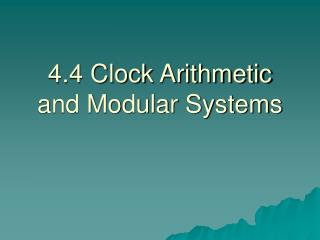# 4.4 Clock Arithmetic and Modular Systems - PowerPoint PPT PresentationDownload Presentation4.4 Clock Arithmetic and Modular Systems

4.4 Clock Arithmetic and Modular Systems
Download Presentation## 4.4 Clock Arithmetic and Modular Systems

- - - - - - - - - - - - - - - - - - - - - - - - - - - E N D - - - - - - - - - - - - - - - - - - - - - - - - - - -
##### Presentation Transcript

1. 4.4 Clock Arithmetic and Modular Systems

2. 12-hour Clock System • Based on an ordinary clock face • 12 replaced with a zero • Minute hand is left off

3. The clock system is FINITE • Also known as CLOSED • You will only get back a clock number no matter what operation you do to it

4. Addition in the clock system • Add by moving the hour had clockwise • Clock arithmetic only uses whole numbers

5. Example 1 • 6 + 3

6. Example 2 • 10 + 7

7. Example 3 • 11 + 4

8. Let’s make a table for clock addition!

9. Closure Property of Clock Addition Defined • If a, b are any clock #s, then a+b is also in the set under addition.

10. Commutative Property of Clock Addition • If a, b are any clock numbers, then a+b = b+a

11. Identity Property of Clock Addition • When an element and the identity are combined, the original element is returned • Ex: a + i = a a is returned, therefore i is the identity element.

12. Subtraction in Clock Arithmetic • Subtraction is possible by going counter clockwise • We will also use the additive inverse

13. Example 4! • 5 - 7

14. Additive Inverse • An element combined with its additive inverse will return the identity • In our number system:

15. Determine 4’s additive inverse in clock arithmetic: • What number combined with 4 will return the identity?

16. Additive Inverse Property of Clock Addition • Every element of the system has an additive inverse • Table:

17. Subtraction of Clock Numbers • If a,b are clock numbers, then the difference, a-b is defined as: a + (-b): where -b is defined as the inverse of b.

18. Example 5! • 5 – 7 • 5 + (-7) • 5 + 5 = 10